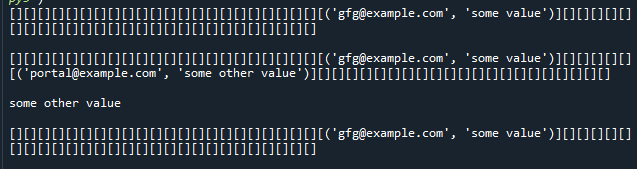# Hash Map in Python

• Difficulty Level : Hard
• Last Updated : 08 Dec, 2020

Hash maps are indexed data structures. A hash map makes use of a hash function to compute an index with a key into an array of buckets or slots. Its value is mapped to the bucket with the corresponding index. The key is unique and immutable. Think of a hash map as a cabinet having drawers with labels for the things stored in them. For example, storing user information- consider email as the key, and we can map values corresponding to that user such as the first name, last name etc to a bucket.

Hash function is the core of implementing a hash map. It takes in the key and translates it to the index of a bucket in the bucket list. Ideal hashing should produce a different index for each key. However, collisions can occur. When hashing gives an existing index, we can simply use a bucket for multiple values by appending a list or by rehashing.

Attention reader! Don’t stop learning now. Get hold of all the important DSA concepts with the DSA Self Paced Course at a student-friendly price and become industry ready.  To complete your preparation from learning a language to DS Algo and many more,  please refer Complete Interview Preparation Course.

In case you wish to attend live classes with experts, please refer DSA Live Classes for Working Professionals and Competitive Programming Live for Students.

In Python, dictionaries are examples of hash maps. We’ll see the implementation of hash map from scratch in order to learn how to build and customize such data structures for optimizing search.

The hash map design will include the following functions:

• set_val(key, value): Inserts a key-value pair into the hash map. If the value already exists in the hash map, update the value.
• get_val(key): Returns the value to which the specified key is mapped, or “No record found” if this map contains no mapping for the key.
• delete_val(key): Removes the mapping for the specific key if the hash map contains the mapping for the key.

Below is the implementation.

## Python3

 `class` `HashTable:`` ` `    ``# Create empty bucket list of given size``    ``def` `__init__(``self``, size):``        ``self``.size ``=` `size``        ``self``.hash_table ``=` `self``.create_buckets()`` ` `    ``def` `create_buckets(``self``):``        ``return` `[[] ``for` `_ ``in` `range``(``self``.size)]`` ` `    ``# Insert values into hash map``    ``def` `set_val(``self``, key, val):``       ` `        ``# Get the index from the key``        ``# using hash function``        ``hashed_key ``=` `hash``(key) ``%` `self``.size``         ` `        ``# Get the bucket corresponding to index``        ``bucket ``=` `self``.hash_table[hashed_key]`` ` `        ``found_key ``=` `False``        ``for` `index, record ``in` `enumerate``(bucket):``            ``record_key, record_val ``=` `record``             ` `            ``# check if the bucket has same key as``            ``# the key to be inserted``            ``if` `record_key ``=``=` `key:``                ``found_key ``=` `True``                ``break`` ` `        ``# If the bucket has same key as the key to be inserted,``        ``# Update the key value``        ``# Otherwise append the new key-value pair to the bucket``        ``if` `found_key:``            ``bucket[index] ``=` `(key, val)``        ``else``:``            ``bucket.append((key, val))`` ` `    ``# Return searched value with specific key``    ``def` `get_val(``self``, key):``       ` `        ``# Get the index from the key using``        ``# hash function``        ``hashed_key ``=` `hash``(key) ``%` `self``.size``         ` `        ``# Get the bucket corresponding to index``        ``bucket ``=` `self``.hash_table[hashed_key]`` ` `        ``found_key ``=` `False``        ``for` `index, record ``in` `enumerate``(bucket):``            ``record_key, record_val ``=` `record``             ` `            ``# check if the bucket has same key as ``            ``# the key being searched``            ``if` `record_key ``=``=` `key:``                ``found_key ``=` `True``                ``break`` ` `        ``# If the bucket has same key as the key being searched,``        ``# Return the value found``        ``# Otherwise indicate there was no record found``        ``if` `found_key:``            ``return` `record_val``        ``else``:``            ``return` `"No record found"`` ` `    ``# Remove a value with specific key``    ``def` `delete_val(``self``, key):``       ` `        ``# Get the index from the key using``        ``# hash function``        ``hashed_key ``=` `hash``(key) ``%` `self``.size``         ` `        ``# Get the bucket corresponding to index``        ``bucket ``=` `self``.hash_table[hashed_key]`` ` `        ``found_key ``=` `False``        ``for` `index, record ``in` `enumerate``(bucket):``            ``record_key, record_val ``=` `record``             ` `            ``# check if the bucket has same key as``            ``# the key to be deleted``            ``if` `record_key ``=``=` `key:``                ``found_key ``=` `True``                ``break``        ``if` `found_key:``            ``bucket.pop(index)``        ``return`` ` `    ``# To print the items of hash map``    ``def` `__str__(``self``):``        ``return` `"".join(``str``(item) ``for` `item ``in` `self``.hash_table)`` ` ` ` `hash_table ``=` `HashTable(``50``)`` ` `# insert some values``hash_table.set_val(``'gfg@example.com'``, ``'some value'``)``print``(hash_table)``print``()`` ` `hash_table.set_val(``'portal@example.com'``, ``'some other value'``)``print``(hash_table)``print``()`` ` `# search/access a record with key``print``(hash_table.get_val(``'portal@example.com'``))``print``()`` ` `# delete or remove a value``hash_table.delete_val(``'portal@example.com'``)``print``(hash_table)`

Output:Time Complexity:

Memory index access takes constant time and hashing takes constant time. Hence, the search complexity of a hash map is also constant time, that is, O(1).

My Personal Notes arrow_drop_up